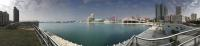#### 此篇文章獻給有緣人自行研究

<XQ操盤高手>

(我有測試，在免費的XQ權限下可以使用)

KD Strategy(Long)，是我擬定的一個KD策略

//--KD Strategy(Long)

input: Length(9), RSVt(3), Kt(3), _stop(5); //參數

SetInputName(1, "計算期數");

SetInputName(2, "RSVt權數");

SetInputName(3, "Kt權數");

SetInputName(4, "停損(%)");

variable: rsv(0), k(0), _d(0);

xf_Stochastic("W",length,RSVt,Kt,rsv,k,_d);

variable:_MarketPosition(0);

if _MarketPosition<>1 and k crosses over _d and K<80 then

begin

_MarketPosition=1; //有部位

value1=close;//估計買入成本

value2=date; //買入那天

end

else

if _MarketPosition=1 and k crosses under _d then

begin

plot2(-1,"Sell");//柱圖

_MarketPosition=0;

end

else

plot2(0,"Sell");

if _MarketPosition=1 and close>value1*(1+_stop/100) then

begin

plot3(1,"Cut gain"); //曲線

_MarketPosition=0;

end

else

plot3(0,"Cut gain");

if _MarketPosition=1 and close<=value1 and date<>value2 then

begin

plot4(-1,"Cut loss"); //曲線

_MarketPosition=0;

end

else

plot4(0,"Cut loss");

//-------------(我是切割線)----//

//-------------(我是切割線)----//

//--Weekly_KD

//可在日頻率下查看週K

input: Length(9), RSVt(3), Kt(3); //參數

SetInputName(1, "計算期數");

SetInputName(2, "RSVt權數");

SetInputName(3, "Kt權數");

variable: rsv(0), k(0), _d(0);

xf_Stochastic("W",length,RSVt,Kt,rsv,k,_d);

plot1(k,"K(%)");

plot2(_d,"D(%)");3 次拍手2019/03/31-數據金
2019/03/31Jun碩

2019/03/17-數據金
2019/03/172019/03/17-數據金

2019/03/17

--:--
--:--
1.0x

2.0x
1.75x
1.5x
1.25x
1.0x
0.75x